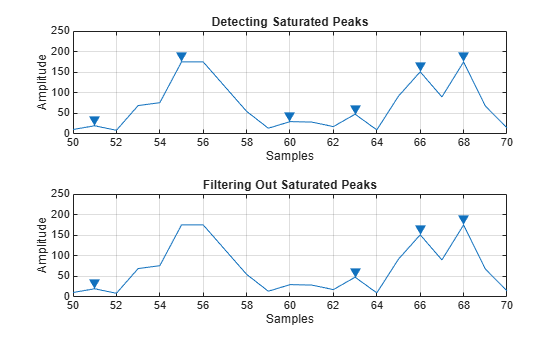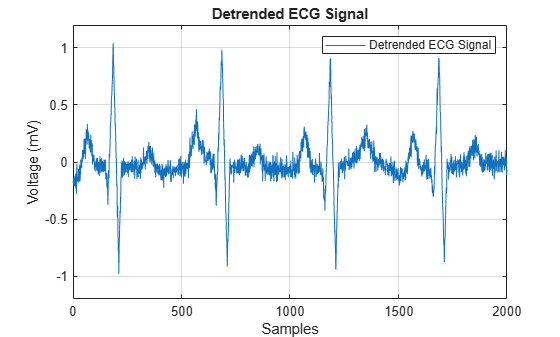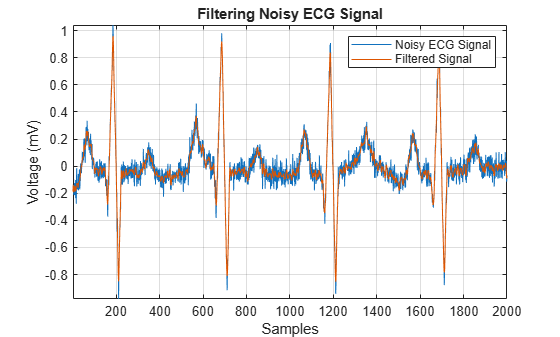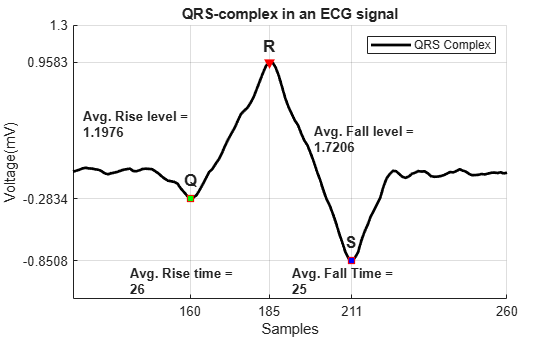Peak Analysis

This example shows how to perform basic peak analysis. It will help you answer questions such as: How do I find peaks in my signal? How do I measure distance between peaks? How do I measure the amplitude of peaks of a signal which is affected by a trend? How do I find peaks in a noisy signal? How do I find local minima?

Finding Maxima or Peaks

The Zurich sunspot relative number measures both the number and size of sunspots. Use the findpeaks function to find the locations and the value of the peaks.

year = sunspot(:,1);
relNums = sunspot(:,2);
findpeaks(relNums,year)
xlabel('Year')
ylabel('Sunspot Number')
title('Find All Peaks')The above plot shows sunspot numbers tabulated over 300 years and labels the detected peaks. The next section shows how to measure distance between these peaks.

Measuring Distance Between Peaks

Peaks in the signal seem to appear at regular intervals. However, some of the peaks are very close to each other. The MinPeakProminence property can be used filter out these peaks. Consider peaks that drop off on both sides by at least 40 relative sunspot numbers before encountering a larger value.

findpeaks(relNums,year,'MinPeakProminence',40)
xlabel('Year')
ylabel('Sunspot Number')
title('Find Prominent Peaks')The following histogram shows the distribution of peak intervals in years:

figure
[pks, locs] = findpeaks(relNums,year,'MinPeakProminence',40);
peakInterval = diff(locs);
histogram(peakInterval)
grid on
xlabel('Year Intervals')
ylabel('Frequency of Occurrence')
title('Histogram of Peak Intervals (years)')AverageDistance_Peaks = mean(diff(locs))
AverageDistance_Peaks = 10.9600

The distribution shows that majority of peak intervals lie between 10 and 12 years indicating the signal has a cyclic nature. Also, the average interval of 10.96 years between the peaks matches the known cyclic sunspot activity of 11 years.

Finding Peaks in Clipped or Saturated Signals

You may want to consider flat peaks as peaks or exclude them. In the latter case, a minimum excursion which is defined as the amplitude difference between a peak and its immediate neighbors is specified using the threshold property.

figure

% Show all peaks in the first plot
ax(1) = subplot(2,1,1);
findpeaks(saturatedData)
xlabel('Samples')
ylabel('Amplitude')
title('Detecting Saturated Peaks')

% Specify a minimum excursion in the second plot
ax(2) = subplot(2,1,2);
findpeaks(saturatedData,'threshold',5)
xlabel('Samples')
ylabel('Amplitude')
title('Filtering Out Saturated Peaks')

% link and zoom in to show the changes
axis(ax,[50 70 0 250])The first subplot shows, that in case of a flat peak, the rising edge is detected as the peak. The second subplot shows that specifying a threshold can help to reject flat peaks.

Measuring Amplitudes of Peaks

This example shows peak analysis in an ECG (Electro-cardiogram) signal. ECG is a measure of electrical activity of the heart over time. The signal is measured by electrodes attached to the skin and is sensitive to disturbances such as power source interference and noises due to movement artifacts.

t = 1:length(noisyECG_withTrend);

figure
plot(t,noisyECG_withTrend)
title('Signal with a Trend')
xlabel('Samples');
ylabel('Voltage(mV)')
legend('Noisy ECG Signal')
grid onDetrending Data

The above signal shows a baseline shift and therefore does not represent the true amplitude. In order to remove the trend, fit a low order polynomial to the signal and use the polynomial to detrend it.

[p,s,mu] = polyfit((1:numel(noisyECG_withTrend))',noisyECG_withTrend,6);
f_y = polyval(p,(1:numel(noisyECG_withTrend))',[],mu);

ECG_data = noisyECG_withTrend - f_y;        % Detrend data

figure
plot(t,ECG_data)
grid on
ax = axis;
axis([ax(1:2) -1.2 1.2])
title('Detrended ECG Signal')
xlabel('Samples')
ylabel('Voltage(mV)')
legend('Detrended ECG Signal')After detrending, find the QRS complex, which is the most prominent repeating peak in the ECG signal. The QRS complex corresponds to the depolarization of the right and left ventricles of the human heart. It can be used to determine a patient's cardiac rate or predict abnormalities in heart function. The following figure shows the shape of the QRS complex in an ECG signal.Thresholding to Find Peaks of Interest

The QRS complex consists of three major components: Q wave, R wave, S wave. The R waves can be detected by thresholding peaks above 0.5 mV. Notice that the R waves are separated by more than 200 samples. Use this information to remove unwanted peaks by specifying a 'MinPeakDistance'.

[~,locs_Rwave] = findpeaks(ECG_data,'MinPeakHeight',0.5,...
'MinPeakDistance',200);

For detection of the S-waves, find the local minima in the signal and apply thresholds appropriately.

Finding Local Minima in Signal

Local minima can be detected by finding peaks on an inverted version of the original signal.

ECG_inverted = -ECG_data;
[~,locs_Swave] = findpeaks(ECG_inverted,'MinPeakHeight',0.5,...
'MinPeakDistance',200);

The following plot shows the R waves and S waves detected in the signal.

figure
hold on
plot(t,ECG_data)
plot(locs_Rwave,ECG_data(locs_Rwave),'rv','MarkerFaceColor','r')
plot(locs_Swave,ECG_data(locs_Swave),'rs','MarkerFaceColor','b')
axis([0 1850 -1.1 1.1])
grid on
legend('ECG Signal','R waves','S waves')
xlabel('Samples')
ylabel('Voltage(mV)')
title('R wave and S wave in Noisy ECG Signal')Next, we try and determine the locations of the Q waves. Thresholding the peaks to locate the Q waves results in detection of unwanted peaks as the Q waves are buried in noise. We filter the signal first and then find the peaks. Savitzky-Golay filtering is used to remove noise in the signal.

smoothECG = sgolayfilt(ECG_data,7,21);

figure
plot(t,ECG_data,'b',t,smoothECG,'r')
grid on
axis tight
xlabel('Samples')
ylabel('Voltage(mV)')
legend('Noisy ECG Signal','Filtered Signal')
title('Filtering Noisy ECG Signal')We perform peak detection on the smooth signal and use logical indexing to find the locations of the Q waves.

[~,min_locs] = findpeaks(-smoothECG,'MinPeakDistance',40);

% Peaks between -0.2mV and -0.5mV
locs_Qwave = min_locs(smoothECG(min_locs)>-0.5 & smoothECG(min_locs)<-0.2);

figure
hold on
plot(t,smoothECG);
plot(locs_Qwave,smoothECG(locs_Qwave),'rs','MarkerFaceColor','g')
plot(locs_Rwave,smoothECG(locs_Rwave),'rv','MarkerFaceColor','r')
plot(locs_Swave,smoothECG(locs_Swave),'rs','MarkerFaceColor','b')
grid on
title('Thresholding Peaks in Signal')
xlabel('Samples')
ylabel('Voltage(mV)')
ax = axis;
axis([0 1850 -1.1 1.1])
legend('Smooth ECG signal','Q wave','R wave','S wave')The above figure shows that the QRS complex successfully detected in the noisy ECG signal.

Error Between Noisy and Smooth Signal

Notice the average difference between the QRS complex in the raw and the detrended filtered signal.

% Values of the Extrema
[val_Qwave, val_Rwave, val_Swave] = deal(smoothECG(locs_Qwave), smoothECG(locs_Rwave), smoothECG(locs_Swave));

meanError_Qwave = mean((noisyECG_withTrend(locs_Qwave) - val_Qwave))
meanError_Qwave = 0.2771
meanError_Rwave = mean((noisyECG_withTrend(locs_Rwave) - val_Rwave))
meanError_Rwave = 0.3476
meanError_Swave = mean((noisyECG_withTrend(locs_Swave) - val_Swave))
meanError_Swave = 0.1844

This demonstrates that it is essential to detrend a noisy signal for efficient peak analysis.

Peak Properties

Some important peak properties involve rise time, fall time, rise level, and fall level. These properties are computed for each of the QRS complexes in the ECG signal. The average values for these properties are displayed on the figure below.

avg_riseTime = mean(locs_Rwave-locs_Qwave); % Average Rise time
avg_fallTime = mean(locs_Swave-locs_Rwave); % Average Fall time
avg_riseLevel = mean(val_Rwave-val_Qwave);  % Average Rise Level
avg_fallLevel = mean(val_Rwave-val_Swave);  % Average Fall Level

helperPeakAnalysisPlot(t,smoothECG,...
locs_Qwave,locs_Rwave,locs_Swave,...
val_Qwave,val_Rwave,val_Swave,...
avg_riseTime,avg_fallTime,...
avg_riseLevel,avg_fallLevel)